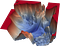# Animations of Gradient Descent and Loss Landscapes of Neural Networks in Python

During my journey of learning various machine learning algorithms, I came across loss landscapes of neural networks with their mountainous terrains, ridges and valleys. These loss landscapes were looking very different from the convex and smooth loss landscapes I encountered with linear and logistic regression. In the following article, we will create loss landscapes of neural networks and animate gradient descent using the MNIST-dataset.Fig. 1: Loss landscape of a convolutional neural network with 56 layers (VGG-56, source¹)

The above image exemplarily depicts the highly non-convex loss landscape of a neural network. A loss landscape is a visual representation of values a cost function takes on for a given range of parameter values given…

# Animations of Logistic Regression with Python

This article is about creating animated plots of simple and multiple logistic regression with batch gradient descent in Python. In the end, I will also present a visual explanation of why the cross-entropy cost function is the method of choice to quantify costs in logistic regression. In terms of structure and content, this article relates to — and partially builds on — previous articles I wrote about creating animations of batch gradient descent with the example of simple linear and multiple linear regression. The general idea is to set up a logistic regression model and train the model on some…

# Animations of Multiple Linear Regression with Python

In this article, we aim to expand our capabilities in visualizing gradient descent to Multiple Linear Regression. This is the follow-up article to “Gradient Descent Animation: 1. Simple linear regression”. Just as we did before, our goal is to set up a model, fit the model to our training data using batch gradient descent while storing the parameter values for each epoch. Afterwards, we can use our stored data to create animations with Python’s celluloid module.

This is the revised version of an article about the same topic I uploaded on July 20th. …

# Gradient Descent animation: 1. Simple linear Regression

## This is the first part of a series of articles on how to create animated plots visualizing gradient descent.

The Gradient Descent method is one of the most widely used parameter optimization algorithms in machine learning today. Python’s celluloid-module enables us to create vivid animations of model parameters and costs during gradient descent.

In this article, I exemplarily want to use simple linear regression to visualize batch gradient descent. The goal is to build a linear regression model and train it on some data points we made up. For every training round (‘epoch’), we intend to store current model parameters and costs. Finally, we aim to create some animations with our stored values.

## Set up the model

There are various articles on how…

# McNemar’s exact test from scratch with Python: Evaluation of SARS-CoV-1 diagnostic test performance

This article is about McNemar’s exact test and its implementation in Python. I want to begin with indications and test theory of McNemar’s exact test before we will address how to perform the test from scratch with Python. We will use real data from the SARS coronavirus epidemic in 2003 as an example. At the end, we want to validate our result by comparing it to the result we obtain with Python’s built-in function.

McNemar’s exact test is a statistical test used for correlated, binary data in 2x2 tables. McNemar’s exact test is the dichotomous equivalent to the paired t-test…

# Pearson’s chi-squared test from scratch with Python

After having discussed Fisher‘s exact test and its implementation with Python in my last article, I now want to dedicate myself to another hypothesis test named after another famous statistician: Pearson‘s chi-squared test.

Once again, I first want to introduce some theoretical aspects of the test before we conduct the test in Python from scratch. At the end, we want to compare our test result to the result we get with Python‘s built-in function.

Pearson’s chi-squared test is a hypothesis test which is used to determine whether there is a significant association between two categorical variables in a contingency table…

# Fisher’s exact test from scratch with Python

In this article, I want to give a brief review of Fisher’s exact test and then continue to show its implementation from scratch with Python. After that, we want to validate our result by comparing it to the output we get from Python’s built-in function.

Sir Ronald Fisher (17 February 1890 - 29 July 1962) was arguably one of the most famous statisticians of the 20th century. One of his greatest contributions to modern statistics was “Fisher’s exact test”.

Fisher’s exact test is used to determine whether there is a significant association between two categorical variables in a contingency table…## Tobias Roeschl

Resident physician passionate about data science, statistics and machine learning. Get in touch: www.linkedin.com/in/tobi-roeschl-60430217b